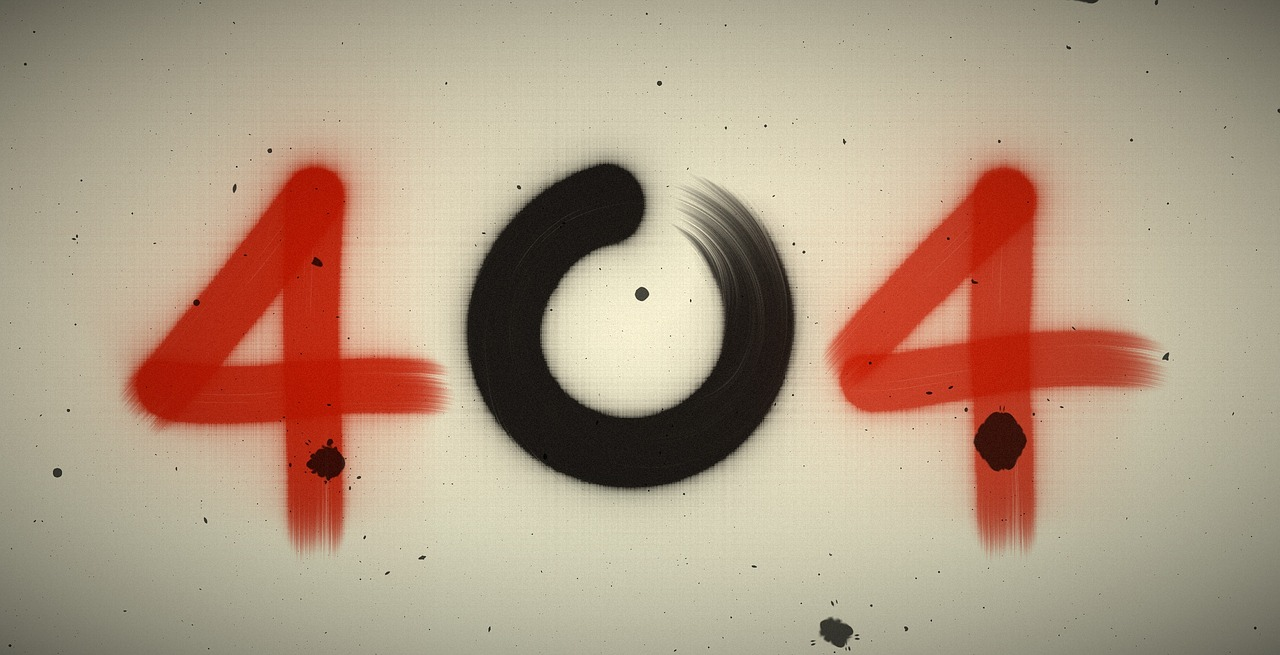Our page on Facebook with over 2 million followers was deleted. Follow the new page here: Physics-Astronomy.com Facebook Page

## Post per Page

So do you think you solve this math problem?: 6^2 ÷ 2(3) + 4 = ? There are actually two ways that I have so far encountered, to solve this problem with the order of operations. Principally, you can multiply the 2 and the 3 together first, or you can either divide 6^2 by 2 before multiplying it by 3. The most public solutions to this problem are 10 and 58. Google calculator and WolframAlpha both give 58 when given this problem. So what answer did you get, and what method did you use to solve it? See the complete clarification of this problem in the video below.